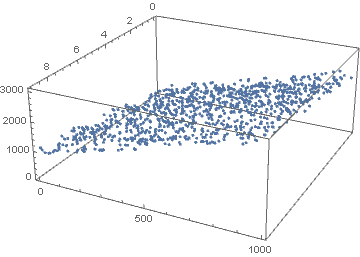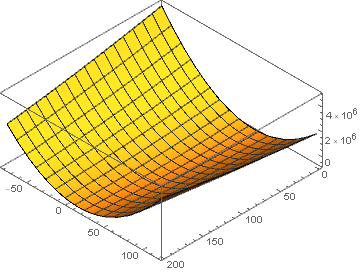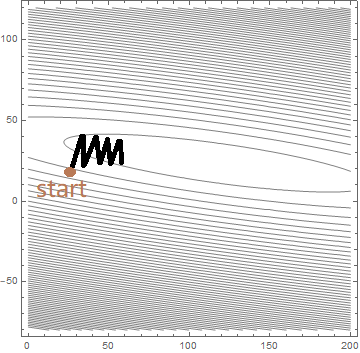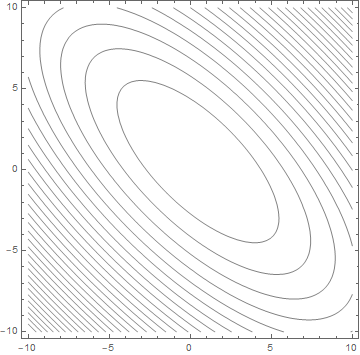Fenrier Lab

# 为何要对数据归一化或标准化

$z(x, y) = 100 x + 20 y$

$\hat{z}(x, y) = \theta_1 x + \theta_2 y$

random = np.random

# 定义平面方程
z = lambda x, y: 100 * x + 20 * y + random.rand(400)

# 生成随机点
n = 1000
x = random.rand(n) * 10
x = x.astype(int)
y = random.rand(n) * 50
y = y.astype(int)

data=pd.DataFrame([x, y]).T
data.columns=["x", "y"]
data["z"] = data.apply(lambda row: z(row, row), axis=1)$l(x_i, y_i, z_i) = \left( z_i - \hat{z}(x_i, y_i) \right)^2$

\begin{aligned} L(\theta_1, \theta_2) &=\frac 1 {2n} \sum_{i=1}^n \left( z_i - \hat{z}(x_i, y_i) \right)^2\\ &=\frac 1 {2n}\sum_{i=1}^n (z_i - \theta_1 x_i - \theta_2 y_i)^2\\ &=\frac 1 {2n}\sum_{i=1}^n (x_i^2\theta_1^2 + y_i^2 \theta_2^2 + 2x_iy_i \theta_1 \theta_2- 2x_iz_i\theta_1 -2y_iz_i \theta_2+ z_i^2) \end{aligned}

$L(\theta_1, \theta_2) = \frac 1 {2n}\left(\sum_{i=1}^n x_i^2 \theta_1^2 + \sum_{i=1}^n y_i^2 \theta_2^2+ 2\sum_{i=1}^n x_i y_i \theta_1 \theta_2 - 2 \sum_{i=1}^nx_i z_i \theta_1 - 2\sum_{i=1}^n y_i z_i \theta_2\right)$$(\theta_1, \theta_2) = \arg \min_{\theta_1, \theta_2} L(\theta_1, \theta_2)$

$\theta^{(n+1)} = \theta^{(n)} - \alpha \frac{\partial L}{\partial \theta}\mid_{\theta = \theta^{(n)}}$$\sum_{i=1}^n x_i^2 = 27995, \quad\sum_{i=1}^n y_i^2 = 787213$

from sklearn.preprocessing import MinMaxScaler
minmax = MinMaxScaler()
minmax.fit(data)
normalize_data=minmax.transform(data)